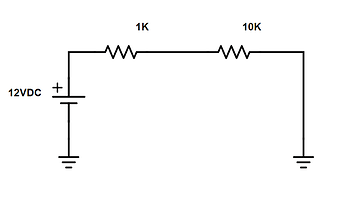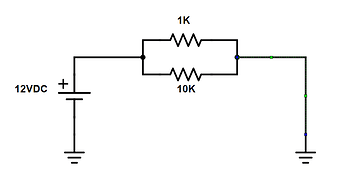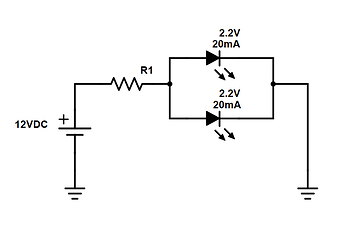# 簡單直流電路中的歐姆定律計算

## 串聯電阻電路I = V / R = 0.00109A

1KΩ 電阻上的壓降為：V = 0.00109 X 1000 = 1.09 V
10KΩ 電阻上的壓降為：V = 0.00109 X 10000 = 10.9 V

## 並聯電阻電路• 1KΩ 電阻上的電流為：I = 12 / 1000 = 0.012A
• 10KΩ 電阻上的電流為：I = 12 / 10000 = 0.0012A
• 電路的總電流為：0.0012A + 0.012A = 0.0132 A

• 串聯電路的功率消耗為：12V x 0.00109A = 0.01308W
• 並聯電路的功率消耗為：12V x 0.0132A = 0.1584W

## 串並聯電路• R = 9.8 / 0.040
R = 245Ω